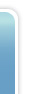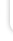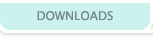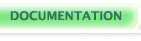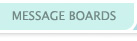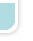## Integers

Integers hold non-fractional numerical values. An "int" can have a value from -2,147,483,648 to 2,147,483,647.

```int an_int; //this creates an int named 'an_int'
int another_int; //this creates another int.

an_int = 5; //this sets it to the value 5.
another_int = 3; //another_int is now equal to 3.

another_int = an_int; //another_int is now equal to 5.

an_int = 7; //an_int is now 7, but another_int is still 5.
```

The verge integer is 32 bit, here's a detailed run down of what that means:
In a 32 bit integer, there are 32 bits than can be either on or off
This is 2^32 combinations, or 4294967296
Binary numbers: 0 1 0 1 1.....0
Equivalent to: 2^0+2^1+2^2+2^3+2^4...-2^31
Which is: 1 + 2 + 4 + 8 + 16....-2147483648
Why is the last number (bit) negative?
So we can store all the negative numbers easily as well.
Then it is still just case of adding to bits to get the number.
Note that as 0 has to be stored as well, there is one less positive than negative
So in a 32 bit int, the number can be between -2^31 and 2^31-1
Alternatively in decimal from -2147483648 to 2147483647
Eg. The number 13 is stored as 8+4+1 or 1011000...0
The number -13 is stored as -2147483648 plus everything BUT 8+4 so 11001111...1
Note that the +1 is still on, as negative numbers stretch one lower than positives
Because the top bit is negative, in an overflow case (trying to store to large a number) you will 'wrap around' and get a negative number.

Talkback

Post a new comment?

Talkback #2 written by RageCage on 2004-09-20.

no. spaces dont generally matter. If you're doing a true or false condition and use == then you want to make sure that the two are right next to each other, otherwise it doesnt matter at all.

Talkback #1 written by gamer83 on 2004-09-16.

I have a question is there a space before and after each equal sign?

Post a new comment?

 Doc Nav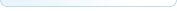Your docs View All Docs If you log in, you can edit the documentation, or create your own documents and tutorials!Ben McGraw's lovingly crafted this website from scratch for years.
It's a lot prettier this go around because of Jon Wofford.
Verge-rpg.com is a member of the lunarnet irc network, and would like to take this opportunity to remind you that regardless how babies taste, it is wrong to eat them.